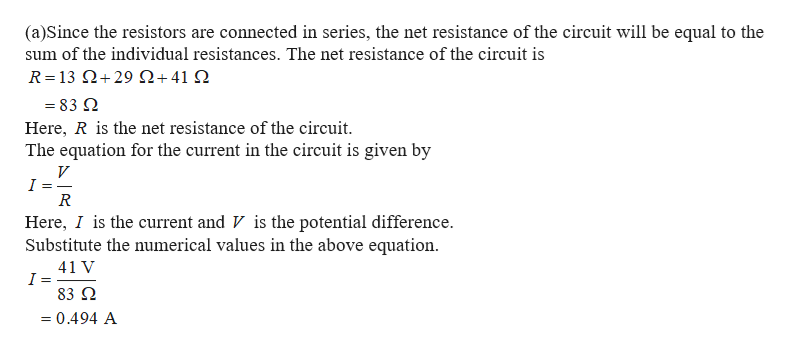# A student in the laboratory connects a 13 Ω resistor, a 29 Ω resistor, and a 41 Ω resistor in series and then connects the arrangement to a 41-V dc source.(a) How much current is in the circuit?___ A(b) How much power is expended in the circuit?___ W

Question
1 views

A student in the laboratory connects a 13 Ω resistor, a 29 Ω resistor, and a 41 Ω resistor in series and then connects the arrangement to a 41-V dc source.

(a) How much current is in the circuit?
___ A

(b) How much power is expended in the circuit?
___ W
check_circle

star
star
star
star
star
1 Rating
Step 1help_outlineImage Transcriptionclose(a)Since the resistors are connected in series, the net resistance of the circuit will be equal to the sum of the individual resistances. The net resistance of the circuit is R= 13 Ω+ 29 Ω+41 Ω =83 Q Here, R is the net resistance of the circuit. The equation for the current in the circuit is given by V I R Here, I is the current and V is the potential difference Substitute the numerical values in the above equation 41 V I 83 Q 0.494 A fullscreen

### Want to see the full answer?

See Solution

#### Want to see this answer and more?

Solutions are written by subject experts who are available 24/7. Questions are typically answered within 1 hour.*

See Solution
*Response times may vary by subject and question.
Tagged in

### Physics